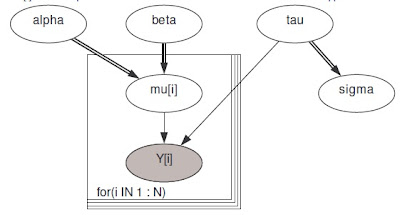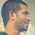## Sunday, May 13, 2012

### Graphical model diagrams in Doing Bayesian Data Analysis versus traditional convention

In this post I contrast conventions for illustrating hierarchical models. On the one hand, there is the traditional convention as used, for example, by DoodleBUGS. On the other hand, there is the style used in Doing Bayesian Data Analysis (DBDA). I explain the advantages of the style in DBDA.

Consider a generic model for Bayesian linear regression. The graphical model diagram in DBDA looks like this:Graphical diagram in Doing Bayesian Data Analysis.
A corresponding graphical model diagram in DoodleBUGS looks something like this:Graphical diagram from DoodleBUGS.
The DoodleBUGS diagrams are much like conventional graphical diagrams used in computer science and statistics.

Which diagram is better for explaining the model? For me, it's the diagrams in DBDA.
• The diagrams in DBDA show at a glance what the distribution is for each variable. By contrast, the diagrams in DoodleBUGS do not show the distributions at all. Instead, you have to cross reference the equations, shown elsewhere.
• The diagrams in DBDA show which parameters "live together" in the same distribution. For example, μi and τ are seen to be the mean and precision of the same normal distribution. By contrast, the diagrams in DoodleBUGS do not show which distributions the parameters "live in". For example, we do not know from the diagram whether μi and τ are in the same distribution or not. To find out, you have to look the equations, shown elsewhere.
• There are other explanatory advantages of the format in DBDA. In particular, the icons of the distributions show directly whether a variable is discrete or continuous, and its range. For example, the icon of the gamma distribution shows that the variable is continuous and has a lower bound. The icon of the Bernoulli distribution (not illustrated here, but repeatedly in the book) shows that the variable has two discrete values. By contrast, conventional diagrams like DoodleBUGS indicate continuous versus discrete by the arbitrary shape of the figure that surrounds the variable: oval for continuous and square for discrete. Or was it oval for discrete and square for continuous? It's easy to get confused by arbitrary conventions.

Which diagram is better for understanding the corresponding JAGS/BUGS model specification? For me, it's the diagrams in DBDA. The key reason is that the diagrams in DBDA have a much more direct correspondence to lines of code in JAGS/BUGS: (Usually) each arrow in the DBDA diagram corresponds to a line of code in the JAGS/BUGS model specificaion. Notice in the DBDA diagram above, there are five arrows. Each arrow has a corresponding line of code in the model specification:
Notice that the DoodleBUGS diagram also has five arrows, but those arrows have no direct correspondence to the model specification! In particular, there is no line of code that says y is related to tau, and a separate line of code that says y is related mu, and a separate line of code that says mu is related to alpha, and another line of code that says mu is related to beta.

The style of diagrams in DBDA are a direct expression of the conceptual distributions and dependencies in the model. And, if you can draw such a picture, it is relatively straightforward to express it in JAGS/BUGS. But the conventional diagrams like DoodleBUGS leave out a huge amount of important conceptual information, and provide little guidance for how to express the model in JAGS/BUGS. Thus, both pedagogically and practically, I prefer the diagrams in DBDA.

One thing that might be improved in the DBDA diagrams is the specification of iteration. In its current form, iteration is indicated ambiguously with an ellipsis that does not indicate explicitly which index is being iterated. In some hierarchical models it can be unclear which index is implied. This could be clarified by using some sort of "plate" notation like what is used in DoodleBUGS, but when plates are drawn in the DBDA diagrams, the overall effect gets visually messy. A simple fix is simply to indicate the index and its limits next to the ellipsis.

#### 15 comments:

1.Have you made code or distribution templates available to create these types of models? If so, can you link to them in the post for easy access?

2.I make the diagrams "by hand" in OpenOffice.

3.I find the diagrams in DBDA to be very clear. These were the first hierarchical model diagrams I had encountered and was a bit dismayed to find that this wasn't the convention.

4.I think the diagrams are great.
I asked a question on Tex.SE about how production of such diagrams could be automated or produced with code.

The answers might interest others:

http://tex.stackexchange.com/q/55869/151

In particular, there's an example of how the diagram could be produced using TikZ.

5.Very interesting to try TikZ! And thanks for posting the question on stackexchange. Exciting as the TikZ approach is, does coding-up a big diagram in TikZ commands really save effort compared to assembling graphical components, WYSIWYG style, in a drawing application? I suppose it would be most exciting if a JAGS/BUGS model specification could be automatically parsed and converted to a diagram -- *that* would be cool. Thanks again.

6.This comment has been removed by the author.

7.I posted a related question on stats.SE about using this approach to visualize results:

http://stats.stackexchange.com/q/31302/1381

In brief: it is not clear to me from this example, but it appears that the curves are 'generic' representations of the distributions that neither represent the prior nor the posterior estimates - of course it would be useless to represent the distributions all as basically flat priors, but my question is this - would it be useful to print the posteriors? And if beta0 were indexed (e.g. for treatment 1...n,), the posteriors could be drawn over each other overlapped. I am about to create such a figure, feedback would be suggested

8.Thanks for your question.

The diagrams are intended to communicate the structure of the prior and likelihood. For that purpose, iconic distributions are better than particular choices of hyperprior constants.

For example, the iconic gamma, with its sharply descending curve on the left, communicates instantly that the distribution is limited on the left but has infinite extent to the right. If instead it showed a gamma(0.01,0.01) or whatever, it would be too easy to visually confuse with an exponential distribution.

Similarly, the iconic beta distribution instantly communicates that the distribution is limited on both ends. If instead it showed an "uninformed" Haldane prior, approximated by beta(0.0001,0.0001), it would be a confusing squarish U-shaped distribution with spikes at the two ends, that might even be visually confused with a Bernoulli distribution.

Thus, the iconic distributions do a good job for their intended purpose.

It would not be appropriate to display the posterior this way because the marginals on the posterior are not necessarily shaped like any particular basic distribution. For example, a gamma prior on a parameter need not yield a gamma-shaped marginal posterior. Moreover, although the priors on the parameters are independent in the JAGS model, the posterior distribution usually has correlations among parameters.

9.I think that the difference in what I was thinking is that, rather than showing the prior to illustrate both M0 and T0, I was thinking about presenting the posterior of M0 and T0, even something like N(M0,T0), but replace the variable names by figures of the distributions for each answer. Would that make more sense?

10.Displaying the marginals of the posterior in a hierarchical diagram might be useful, but it is far less useful than displaying the prior and it could also be misleading. It's less useful because it no longer maps onto JAGS/BUGS code. It's potentially misleading because the diagrams with separate arrows from marginals suggest independence of those parameters in the joint distribution, but in general the parameters will not necessarily be independent in the posterior. Also, the posterior is often examined from many perspectives other than just the marginals on the single parameters. So, I think the cons outweigh the pros in this case...

11.I really like the DBDA style diagrams! In order to make it easier to draw such diagrams I made a short script that generates distribution plots that can be used as the "building blocks" of a hierarchical model diagram. Sample plots, the script and examples are available here. Any feedback is very appreciated, for example, I was very unsure about the "canonical" shape of many of the distributions.

/Rasmus Bååth

12.Dear Rasmus:

Very nice! Thanks for doing this and thanks for letting us know.

A few comments from after only a quick look:
* For the discrete distributions such as binomial, negative binomial, and so on, it would be better for the bars to be narrower so that bars look distinctly discrete, and the distribution does not look like a histogram over a continuous variable.
* When I display the binomial in a new window from RStudio (on a Windows machine), the semi-transparent rectangle behind "binomial" has a black border. Can that be removed?

Again, thanks for doing this. I hope others will comment and support your efforts!

13.Thanks for the feedback. I made the bars narrower and I fixed the black border bug. I also made
a new thread on the forum if people have more suggestions/find more bugs.

/Rasmus

14.Rasmus:

I posted the message below in the Nabble discussion forum, but thought I'd duplicate it here as a comment, too, just to be sure you (and others) see it:

This is a terrific tool for helping to make attractive DBDA-style diagrams. Thanks very much for posting it!

One way to make creation of diagrams much easier in LibreOffice is to use "glue points" on iconic distributions and equations, with "connectors" between the glue points. That way it is easy to rearrange the distributions with the connecting arrows remaining attached to the appropriate points in the icons. To do this, we have to manually add glue points to the iconic distributions in LibreOffice. While this is not terribly difficult, it is a bit inexact.

So, I am wondering: Is there a way in R to produce the iconic distributions, perhaps in SVG format, that already includes glue points for LibreOffice at the top of each label in the in the graph? I do not know the SVG graphics language and do not know whether it includes specification of glue points, or if instead glue points are specific to LibreOffice.

Thanks again!

15.Hey, what is the diagram software you used to create these dagrams? It is creately ?